# Irreducible element not implies prime

## Statement

An irreducible element in an integral domain need not be a prime element.

## Related facts

An integral domain in which every irreducible is prime is an integral domain where irreducible elements are all prime. Such integral domains are very common. In fact:

## Proof

### Example of a quadratic integer ring

Consider the ring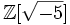$\mathbb{Z}[\sqrt{-5}]$. In this ring, we have: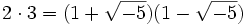$2 \cdot 3 = (1 + \sqrt{-5})(1 - \sqrt{-5})$.

Thus,$2 | (1 + \sqrt{-5})(1 - \sqrt{-5})$, but$2$ does not divide either factor, so$2$ is not prime.

On the other hand,$2$ is irreducible, as can be verified using the algebraic norm. If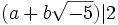$(a + b\sqrt{-5}) | 2$, then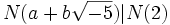$N(a + b \sqrt{-5}) | N(2)$, yielding:$a^2 + 5b^2 | 4$.

But the only possibilities for this are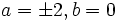$a = \pm 2, b = 0$ or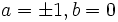$a = \pm 1, b = 0$.

Note that this ring is a Dedekind domain.

### Example of a ring of integer-valued polynomials

Further information: ring of integer-valued polynomials over rational integers

Let$R$ be the ring of integer-valued polynomials over rational integers: this is the ring of those polynomials in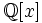$\mathbb{Q}[x]$ that send integers to integers. Then, any binomial polynomial, i.e., any polynomial of the form:$\binom{x}{r} = \frac{x(x-1) \dots (x-r+1)}{r!}$

is irreducible but not prime in$R$.

Unlike the previous example, the ring is not a Noetherian ring.